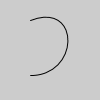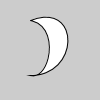Name

### bezierVertex()

Examples```noFill()
beginShape()
vertex(30, 20)
bezierVertex(80, 0, 80, 75, 30, 75)
endShape()
``````beginShape()
vertex(30, 20)
bezierVertex(80, 0, 80, 75, 30, 75)
bezierVertex(50, 80, 60, 25, 30, 20)
endShape()
```
Description Specifies vertex coordinates for Bezier curves. Each call to bezierVertex() defines the position of two control points and one anchor point of a Bezier curve, adding a new segment to a line or shape. The first time bezierVertex() is used within a beginShape() call, it must be prefaced with a call to vertex() to set the first anchor point. This function must be used between beginShape() and endShape() and only when there is no MODE parameter specified to beginShape(). Using the 3D version requires rendering with P3D (see the Environment reference for more information).
Syntax
```bezierVertex(x2, y2, x3, y3, x4, y4)
bezierVertex(x2, y2, z2, x3, y3, z3, x4, y4, z4)
```
Parameters
x2 float: the x-coordinate of the 1st control point float: the y-coordinate of the 1st control point float: the z-coordinate of the 1st control point float: the x-coordinate of the 2nd control point float: the y-coordinate of the 2nd control point float: the z-coordinate of the 2nd control point float: the x-coordinate of the anchor point float: the y-coordinate of the anchor point float: the z-coordinate of the anchor point
Related curveVertex()
vertex()